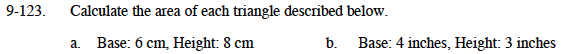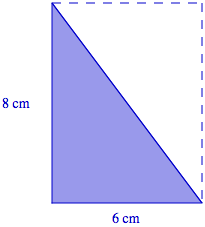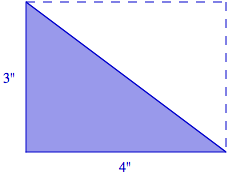### Home > MC1 > Chapter 9 > Lesson 9.3.1 > Problem9-123

9-123.Find the area of the rectangle. Area = base (height)
Then use that area to find the area of the triangle.Find the area of the triangle. Area = base (height)
Then use that area to find the area of the triangle.6 square inches